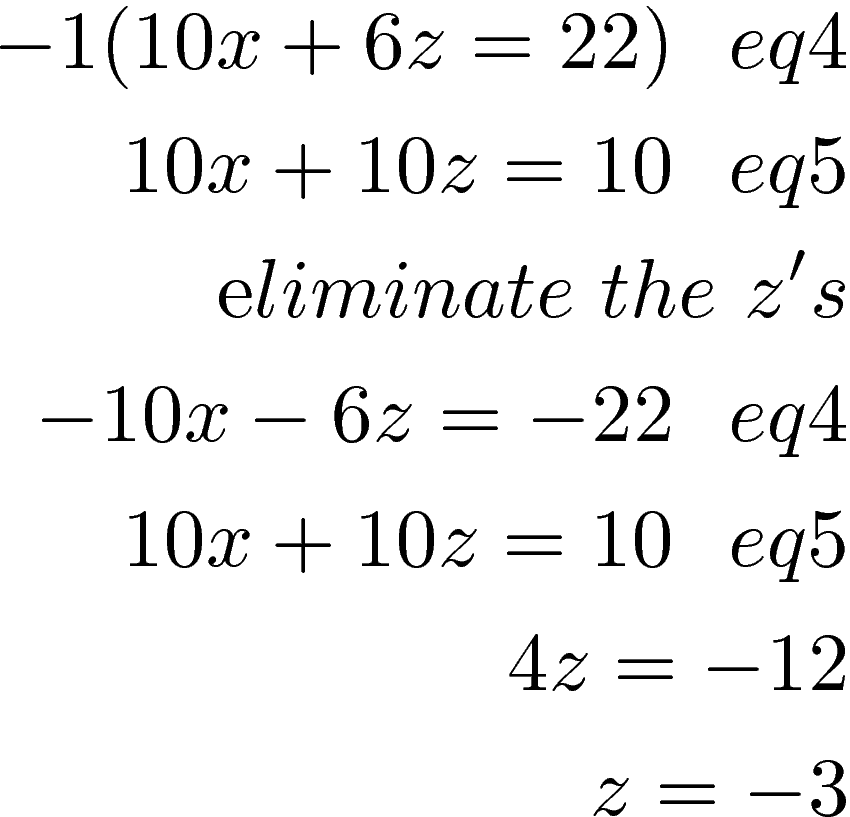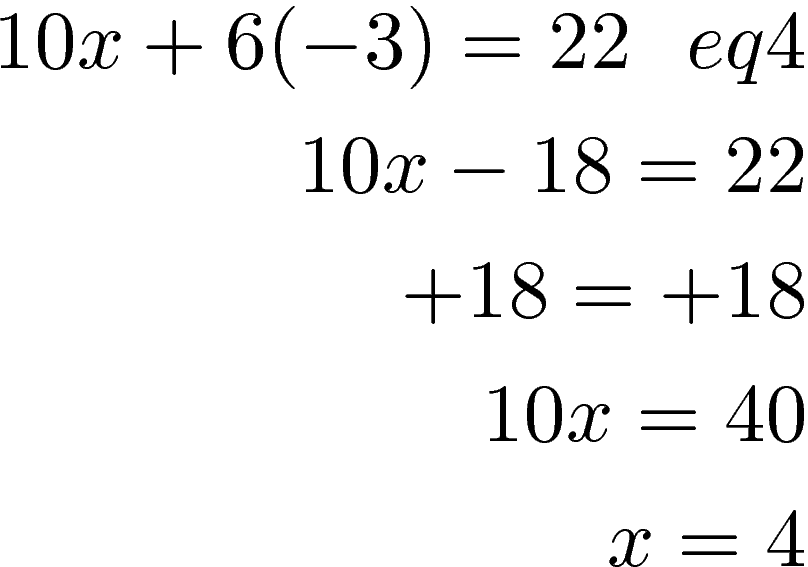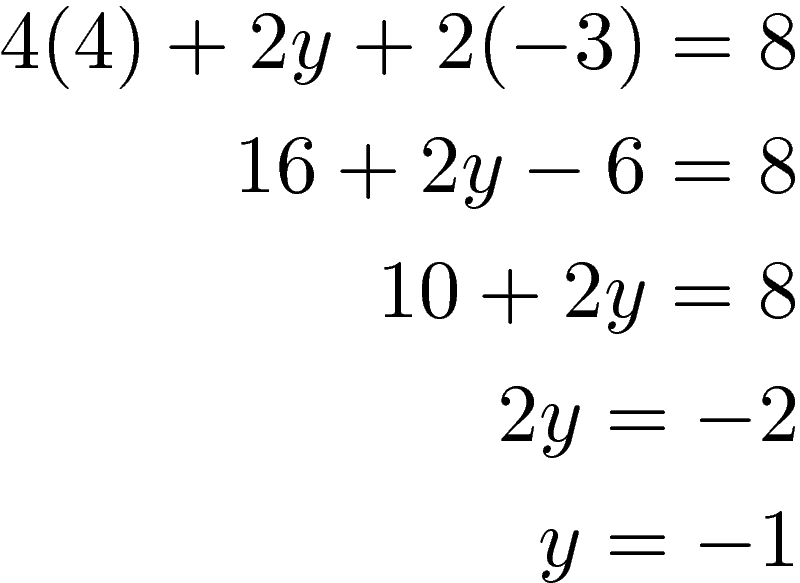# Solving a System of Equations with Three Variables

A system of equations can be solved involving three variables. There are several different ways to accomplish this when three variables are involved. In this post, we will focus on the use of the elimination method.

Our initial system of equations is belowThe values eq1,eq2 and eq3 just mean equation 1, 2, 3

To solve this system we need to first solve two equations as a system and create a fourth equation we will call eq4. We then take eq1 and eq3 to create a new system of equations that creates eq5.It is important to note that for the first two two-variable system of equations you create that you eliminate the same variable it both systems. So fare our example when we take equation 1 and 2 to create equation 4 and then take equation 1 and 3 create equation 5 we must solve for y in both situations or else we will have problems. In addition, you must make sure that all three equations appear at least once in the two two-variable systems of equations. For our purpose, we will use eq1 twice and eq2 and eq3 once.

Eq4 and eq5 are used to find the actual values we need for all three variables. This will make more sense as we go through the example. Therefore, we are going to solve first for y for eq1 and eq2.To eliminate y we need to multiple eq2 by 2 and then combine the equations. Below is the process and the new eq 4We will come back to eq4. For now, we will create eq 5 by eliminating y from eq1 and eq3.We are essentially done using equations 1, 2, and 3. They will not reappear until the end. We will now use equations 4 and 5 to find our answers for two of the three variables.

We now will use eq 4 and 5 to eliminate the variable x. Eliminating x will allow us to solve for z. Doing means we will multiply eq4 by -1.We know z = -3 we can plug this value into either eq4 or 5 to find the answer for x.Now that we know x and z we can plug the two numbers into one of the three original equations to find the value for y. Notice how the first variable we eliminated becomes the last one we solve for.We now know all three values which are

(4, -1, -3)

What this means is that if we were to graph this three equations they would intersect at (4, -1, -3). A solving a system of equations is simply telling us where the lines of the equations intersect.

Conclusion

Solving a system of equations involving three variable is an extension of the two variable system that has already been covered. It provides a mathematician with a tool for solving for more unknown variables. There are practical applications of this as we shall see in the future,